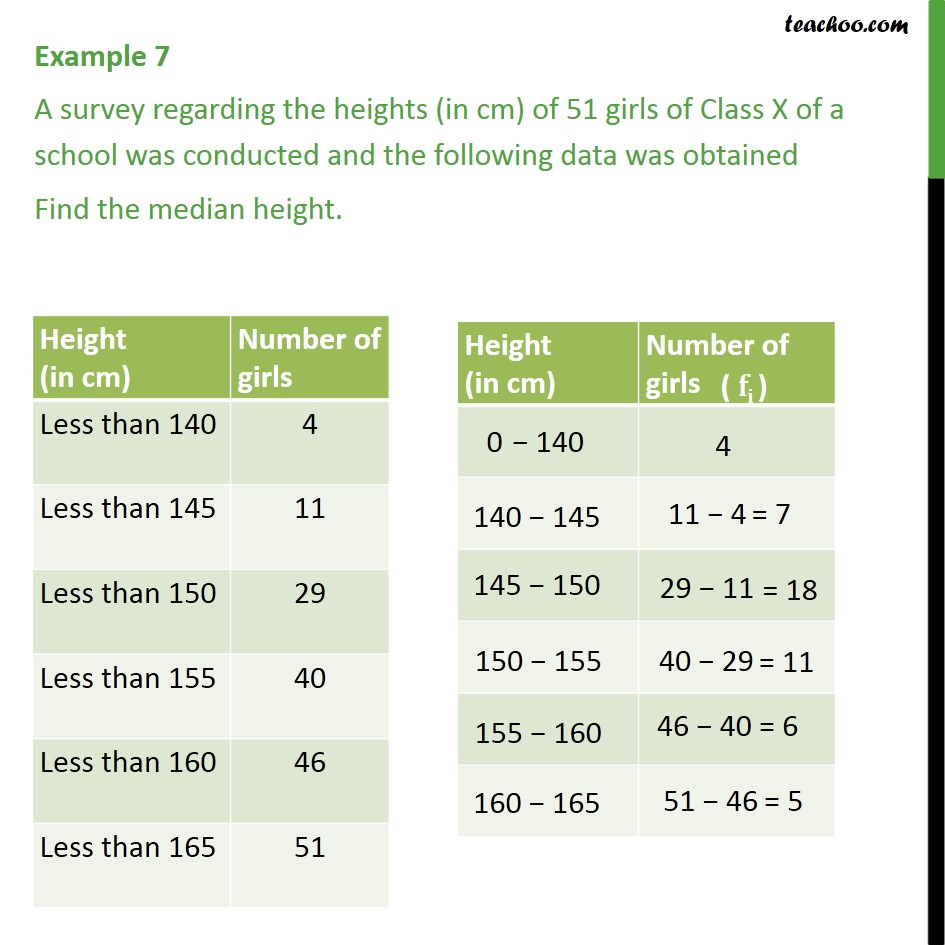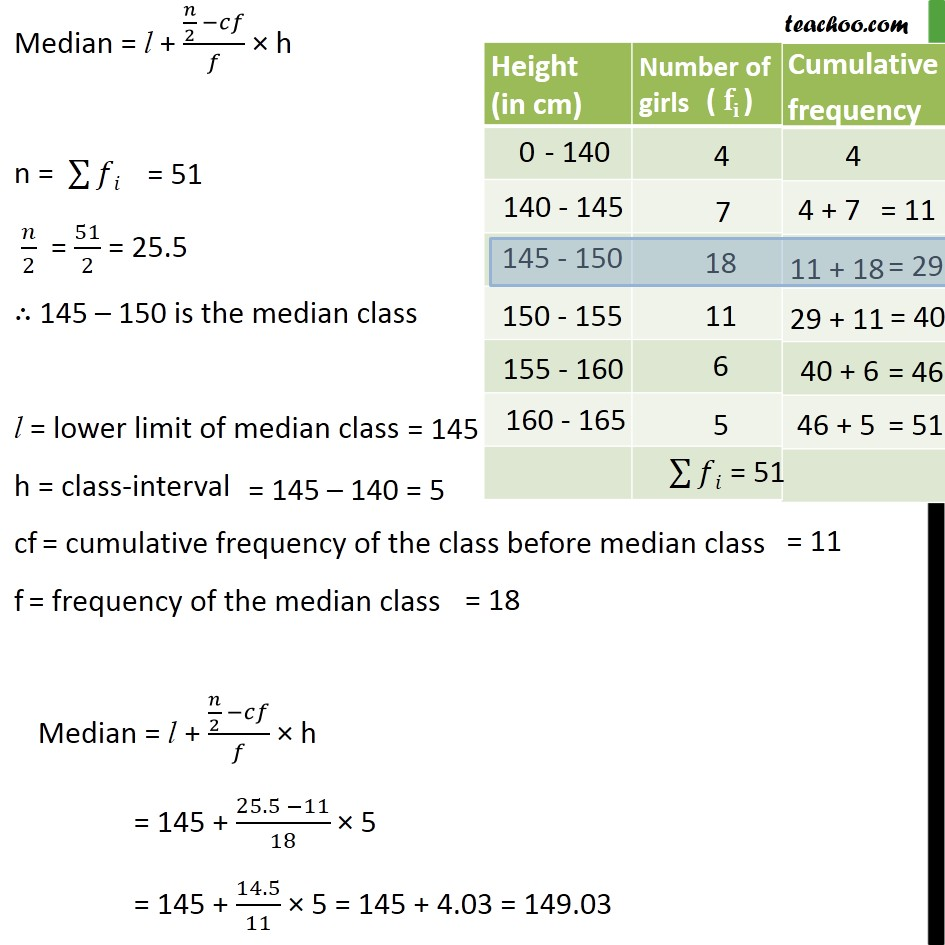1. Class 10
2. Important Questions for Exam - Class 10
3. Chapter 14 Class 10 Statistics

Transcript

Example 7 A survey regarding the heights (in cm) of 51 girls of Class X of a school was conducted and the following data was obtained. Find the median height. Class interval Number of students 10 – 25 2 25 – 40 3 40 – 55 7 55 – 70 6 70 – 85 6 85 – 100 6 Height (in cm) 0 − 140 140 − 145 145 − 150 150 − 155 155 − 160 160 − 165 Number of girls( fi ) 4 11 − 4=7 29 − 11=18 40 − 29=11 46 − 40=6 51 − 46=5 Median = l + (𝑛/2 −𝑐𝑓)/𝑓 × h n = ∑▒𝑓𝑖 = 51 𝑛/2 = 51/2 = 25.5 ∴ 145 – 150 is the median class l = lower limit of median class= 145 h = class-interval= 145 – 140 = 5 cf = cumulative frequency of the class before median class=11 f = frequency of the median class =18 Median = l + (𝑛/2 −𝑐𝑓)/𝑓 × h = 145 + (25.5 −11)/18 × 5 = 145 + 14.5/11 × 5 = 145 + 4.03 = 149.03

Chapter 14 Class 10 Statistics

Class 10
Important Questions for Exam - Class 10